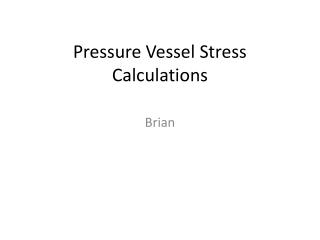DownloadDownload PresentationPressure Vessel Stress Calculations

# Pressure Vessel Stress Calculations

Download Presentation## Pressure Vessel Stress Calculations

- - - - - - - - - - - - - - - - - - - - - - - - - - - E N D - - - - - - - - - - - - - - - - - - - - - - - - - - -
##### Presentation Transcript

1. Stress calculations for a 5 foot Long 2 foot in diameter cylindrical pressure vessel during normal operation pressure of 150 psi • Hoop Stress or Stress in the Circumferential Direction = Pr/t • With P = 150 psi, r = 12 in, and t = .25 in Hoop Stress = 7200 psi • Axial Stress = Pr/2t • With P = 150 psi, r = 12 in, and t = .25 in Axial Stress = 3600 psi

2. Max pressure calculations for a 5 foot Long 2 foot in diameter cylindrical pressure vessel • Calculations based on Grade B pipe with a Minimum Yield Strength: 35000 psi t = .25 in r = 12 in • Max Pressure based on Hoop Stress = 35000 psi Pressure = 729.17 psi • Max Pressure based on Axial Stress = 35000 psi Pressure = 1458.3 psi

3. Stress calculations for 12 in diameter spherical end caps during normal operation pressure of 150 psi. • Hoop Stress = Axial Stress = Pr/2t • With P = 150 psi, r = 6 in, and t = .25 in Hoop Stress = 1800 psi

4. Max pressure calculations for 12 in diameter end caps • Calculations based on Grade b pipe with a Minimum Yield Strength: 35000 psi t = .25 in r = 6 in • Max Pressure based on Hoop Stress = 35000 psi Max Pressure = 2916.7 psi

5. Results • Based on the previous calculations we have determined that the limiting factor in regard to max pressure within the pressure vessel is the Hoop Stress for the cylindrical pressure chamber. But it is still 3.86 times the intended operation pressure of 150 psi.

6. Heating System Selection

7. Convection Incoloy Tubular Heating selected • Is most common in commercial autoclaves • Easily obtained – used in home appliances

8. Insulation of Vessel • Prevent heat from escaping (minimize power required to heat) • Keep surface temperature low to prevent burning danger • Can also add outer covering, i.e. sheet metal (extra cost)

9. Insulation Thickness • 0.4 m O.D. corresponds to a 3.5” insulation layer • Common (R13) • No real gains if any thicker

10. Heating of Vessel • Surface temp. at 3.5” thickness is 80F • Heat loss also levels off at 3.5”

11. Total power to maintain max. temp: ~470W • Total power to heat up air at 15F/min (total 27 min): ~1.5kW • Allowing for thermal mass of parts/molds/pipe, ~4-5 kW needed • Can easily be run on 240V power • Elements can be obtained to provide this • Blowers inside vessel will help maintain uniform temp.

12. Estimated Heating System Costs • 63 ft^2 R13 fiberglass (\$20) • 4-5kW incoloy tubular heating element (\$250) • Potential sheet metal covering (\$125) • Shipping, fittings, etc (\$125 est.) • Subsystem estimated total: \$400-\$500